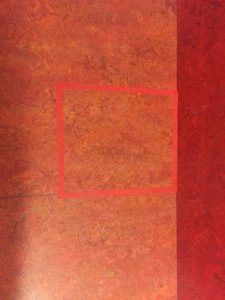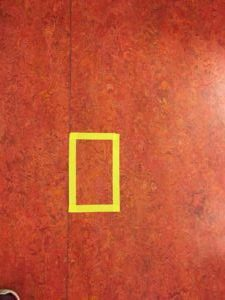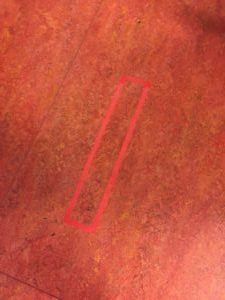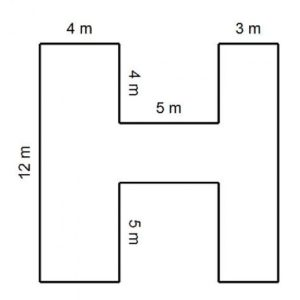# Maths: Area and Perimeter

I’ve decided to do a few blogposts on various areas of the Maths curriculum – sharing some ideas that I use in my classroom. I love teaching Maths, I try to make it as practical as possible – some concepts lend themselves to practical hands on tasks and lessons, while others need lots of drills and practice in copies. The first concept I’ll focus on is Perimeter and Area as these are the concepts we are currently learning about in class.

## What is area? What is perimeter? What’s the difference?

Draw a rectangle on the board. Ask the children to label the perimeter and the area. I explain to the children that to find the perimeter I walk around the shape and to find the area I’m looking at the space between the walls/sides of the rectangle.This image explains both area and perimeter. (Source: TES)

## Squared paper

5 minutes: Get the children to draw different squares and rectangles on the squared paper. Ask them to draw at least 5 different squares/rectangles. Then ask the children to calculate the perimeter of each one. Remind them to walk around the shape (adding the lengths of each side together). Next ask the children to show where the area of each shape is and how they will calculate the area – count the squares. Following this, ask the children if they can spot another way to calculate the area and introduce the concept of length x width. (This will have to be used when there is no squared paper available.)

## Find the area

• Draw and label the lengths of various squares and rectangles on the whiteboard. Ask children to calculate the area. (Use squared paper and blank paper – get the children to draw their own shapes on plain paper and then calculate the area – good to practice drawing lines of varying length)
• Use tape to create squares and rectangles of various sizes around the classroom – in groups, the children can go to each shape, measure the length of each line and then calculate the area of them.
• Calculate the area of the classroom.
• Look at house plans – estimate the size of the rooms and calculate the area.## Name area

Children draw their name in block letters on squared paper. They can then colour in each letter and calculate the area.## Animal area

Love this idea from Muinteoir Fabulous.

View this post on Instagram

A post shared by Muinteoir Fabulous (@muinteoirfabulous) on

## Find the area of compound shapesThese can be difficult for children to calculate. Ask the children to divide the shape into 2/3 rectangles or squares and to find the length of the other sides by using the information they have.

I ask children to label each part (I usually ask them to give each section a letter). It can be a good idea to focus on one section at a time and block out the other sections. I also find a dotted line can help the children to break the shape into manageable sections.

## Interior Designers

Divide the children into groups of 3 or 4 and give them a large sheet of paper (squared or plain). Give each group an area and invite them to choose the shape of the room – will it be a square/rectangle or will it be a compound shape?Each group will be given a certain area for their room and using argos catalogues they can then chose items of furniture that will fit well into their rooms. Most items have the measurements included so children will have to calculate the area of their chosen items to make sure that they will fit in their room. They can then cut out the picture and place it onto the page.

Overall area is a great topic for lots of practical activities and using real life maths (house plans/brochures).

SaveSave

SaveSaveSaveSave

SaveSaveSaveSave

SaveSave

SaveSave

SaveSaveSaveSave

SaveSave

This site uses Akismet to reduce spam. Learn how your comment data is processed.